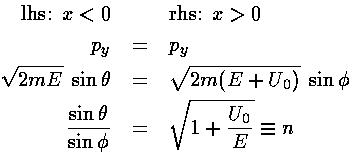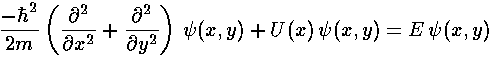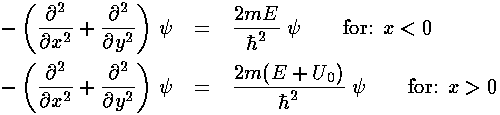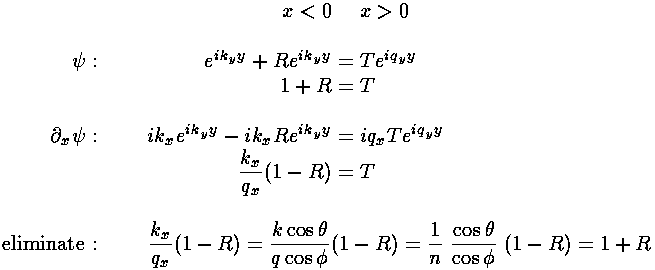## Square Wells p.10

### Reflection from a Two Dimensional Step

We consider next the unbound 2-d step potential. We begin with a simple step potential: for x<0 U=0 and for x>0 U=-U0. We employ the language of light reflecting/refracting off of a semi-infinite sheet of glass. (We avoid the very interesting physics of multiple reflection from a finite thickness of glass...an etalon.)Classically, the downward step in potential at x=0 corresponds to an infinite force in the x direction located just on the x=0 line. Everywhere except x=0 the potential is flat, and so we are almost always in a no-force (i.e., "free particle") situation. Particles move with the speed required for their energy. On the left hand side:

p2=2mE

and on the right hand side we have a faster speed:

p2=2m(E+U0)

Since there is no force in the y direction, the momentum in the y direction: py is conserved, even at the potential step. Thus when a particle makes the energy jump at x=0, px must increase to supply the increase in kinetic energy. Since px is enlarged and py is conserved, the direction of the particle changes. We find:We see that the particle is Snell's Law "refracted" as if with an "index-of-refraction" n. Newton used such a particle-based explanation for the behavior of light. We note some oddities:

1. Moving into a more "optically dense" material (i.e., n>1, like from air to glass) the particle increases in speed. For light, we find the speed of light is slower in glass than in air.

2. The "index-of-refraction" n depends on the particle's energy: higher energy corresponds to smaller n. For visible light in most materials, higher energy means larger n

3. A step up in potential (e.g., U0<0) corresponds to n<1. For light that would be like a transition from glass to air.

4. Classically we have no reflection. As I commented before, even in the WKB approximation there is no reflection; reflection is a result of a large change in potential in a wavelength or so of the wave.

Schrödinger's equation for our problem is:Thus:with solutions:We note that Schrödinger's equation does not require, say, qx, to be a real number, only that qx2+qy2 have a given value. If qx is imaginary, we can have exponentially decaying solutions like: exp(-|qx|x).

Note that since we no longer have a finite sized box we've lost our length scale. We are forced to use dimensioned coordinates.

We now consider a solution that includes incident, reflected, and refracted waves:As usual we must matchandalong the boundary x=0. Thus we end up considering the values offor r=(0,y) and produce functions like:

exp(ikyy) and exp(iqyy)

If ky does not equal qy we would have different wavelengths on each side of the boundary so while we could match at a point, there would be no hope of matching all along the boundary. Thus we immediately produce the "refraction" condition that:

ky=qy

We now proceed to matchandand derive the reflected amplitude R:Since:We can simplify our expressions for R:We note that quantum mechanical reflection is exactly the same as the reflection of TE polarized light. Note the obvious check that here is no reflection if n=1. At normal incidence (=0) R=(1-n)/(1+n). At grazing incidence (=90°) R=-1. If n<1 (i.e., U0<0) we can have total internal reflection for sin>n. As we show below, we have produced an imaginary qx, so the "transmitted" wave does not propagate, rather it decays away for positive x: